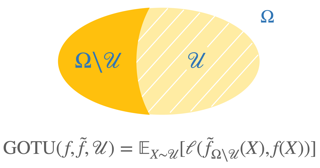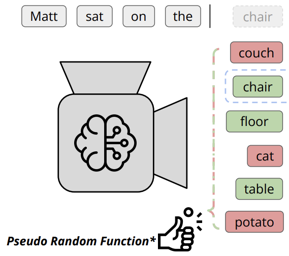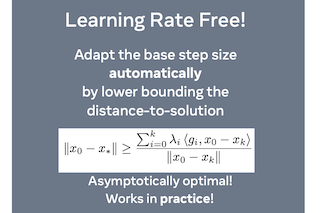Test of Time Award Test Of Time Richard Zemel · Yu Wu · Kevin Swersky · Toniann Pitassi · Cynthia Dwork [ Exhibit Hall 2 ] We propose a learning algorithm for fair classification that achieves both group fairness (the proportion of members in a protected group receiving positive classification is identical to the proportion in the population as a whole), and individual fairness (similar individuals should be treated similarly). We formulate fairness as an optimization problem of finding a good representation of the data with two competing goals: to encode the data as well as possible, while simultaneously obfuscating any information about membership in the protected group. We show positive results of our algorithm relative to other known techniques, on three datasets. Moreover, we demonstrate several advantages to our approach. First, our intermediate representation can be used for other classification tasks (i.e., transfer learning is possible); secondly, we take a step toward learning a distance metric which can find important dimensions of the data for classification. Outstanding Paper Oral Côme Fiegel · Pierre Menard · Tadashi Kozuno · Remi Munos · Vianney Perchet · Michal Valko [ Ballroom A ]Imperfect information games (IIG) are games in which each player only partially observes the current game state. We study how to learn $\epsilon$-optimal strategies in a zero-sum IIG through self-play with trajectory feedback. We give a problem-independent lower bound $\widetilde{\mathcal{O}}(H(A_{\mathcal{X}}+B_{\mathcal{Y}})/\epsilon^2)$ on the required number of realizations to learn these strategies with high probability, where $H$ is the length of the game, $A_{\mathcal{X}}$ and $B_{\mathcal{Y}}$ are the total number of actions for the two players. We also propose two Follow the Regularized leader (FTRL) algorithms for this setting: Balanced FTRL which matches this lower bound, but requires the knowledge of the information set structure beforehand to define the regularization; and Adaptive FTRL which needs $\widetilde{\mathcal{O}}(H^2(A_{\mathcal{X}}+B_{\mathcal{Y}})/\epsilon^2)$ realizations without this requirement by progressively adapting the regularization to the observations. Outstanding Paper Poster Emmanuel Abbe · Samy Bengio · Aryo Lotfi · Kevin Rizk [ Exhibit Hall 1 ]This paper considers the learning of logical (Boolean) functions with focus on the generalization on the unseen (GOTU) setting, a strong case of out-of-distribution generalization. This is motivated by the fact that the rich combinatorial nature of data in certain reasoning tasks (e.g., arithmetic/logic) makes representative data sampling challenging, and learning successfully under GOTU gives a first vignette of an 'extrapolating' or 'reasoning' learner. We then study how different network architectures trained by (S)GD perform under GOTU and provide both theoretical and experimental evidence that for a class of network models including instances of Transformers, random features models, and diagonal linear networks, a min-degree-interpolator is learned on the unseen. We also provide evidence that other instances with larger learning rates or mean-field networks reach leaky min-degree solutions. These findings lead to two implications: (1) we provide an explanation to the length generalization problem (e.g., Anil et al. 2022); (2) we introduce a curriculum learning algorithm called Degree-Curriculum that learns monomials more efficiently by incrementing supports. Outstanding Paper Oral John Kirchenbauer · Jonas Geiping · Yuxin Wen · Jonathan Katz · Ian Miers · Tom Goldstein [ Ballroom C ]Potential harms of large language models can be mitigated by watermarking model output, i.e., embedding signals into generated text that are invisible to humans but algorithmically detectable from a short span of tokens. We propose a watermarking framework for proprietary language models. The watermark can be embedded with negligible impact on text quality, and can be detected using an efficient open-source algorithm without access to the language model API or parameters. The watermark works by selecting a randomized set of "green" tokens before a word is generated, and then softly promoting use of green tokens during sampling. We propose a statistical test for detecting the watermark with interpretable p-values, and derive an information-theoretic framework for analyzing the sensitivity of the watermark. We test the watermark using a multi-billion parameter model from the Open Pretrained Transformer (OPT) family, and discuss robustness and security. Outstanding Paper Poster Aaron Defazio · Konstantin Mishchenko [ Exhibit Hall 1 ]The speed of gradient descent for convex Lipschitz functions is highly dependent on the choice of learning rate. Setting the learning rate to achieve the optimal convergence rate requires knowing the distance D from the initial point to the solution set. In this work, we describe a single-loop method, with no back-tracking or line searches, which does not require knowledge of D yet asymptotically achieves the optimal rate of convergence for the complexity class of convex Lipschitz functions. Our approach is the first parameter-free method for this class without additional multiplicative log factors in the convergence rate. We present extensive experiments for SGD and Adam variants of our method, where the method automatically matches hand-tuned learning rates across more than a dozen diverse machine learning problems, including large-scale vision and language problems. Our method is practical, efficient and requires no additional function value or gradient evaluations each step. An implementation is provided in the supplementary material. Outstanding Paper Oral Aaron Defazio · Konstantin Mishchenko [ Ballroom B ]The speed of gradient descent for convex Lipschitz functions is highly dependent on the choice of learning rate. Setting the learning rate to achieve the optimal convergence rate requires knowing the distance D from the initial point to the solution set. In this work, we describe a single-loop method, with no back-tracking or line searches, which does not require knowledge of D yet asymptotically achieves the optimal rate of convergence for the complexity class of convex Lipschitz functions. Our approach is the first parameter-free method for this class without additional multiplicative log factors in the convergence rate. We present extensive experiments for SGD and Adam variants of our method, where the method automatically matches hand-tuned learning rates across more than a dozen diverse machine learning problems, including large-scale vision and language problems. Our method is practical, efficient and requires no additional function value or gradient evaluations each step. An implementation is provided in the supplementary material. Outstanding Paper Poster Côme Fiegel · Pierre Menard · Tadashi Kozuno · Remi Munos · Vianney Perchet · Michal Valko [ Exhibit Hall 1 ]Imperfect information games (IIG) are games in which each player only partially observes the current game state. We study how to learn $\epsilon$-optimal strategies in a zero-sum IIG through self-play with trajectory feedback. We give a problem-independent lower bound $\widetilde{\mathcal{O}}(H(A_{\mathcal{X}}+B_{\mathcal{Y}})/\epsilon^2)$ on the required number of realizations to learn these strategies with high probability, where $H$ is the length of the game, $A_{\mathcal{X}}$ and $B_{\mathcal{Y}}$ are the total number of actions for the two players. We also propose two Follow the Regularized leader (FTRL) algorithms for this setting: Balanced FTRL which matches this lower bound, but requires the knowledge of the information set structure beforehand to define the regularization; and Adaptive FTRL which needs $\widetilde{\mathcal{O}}(H^2(A_{\mathcal{X}}+B_{\mathcal{Y}})/\epsilon^2)$ realizations without this requirement by progressively adapting the regularization to the observations. Outstanding Paper Oral Emmanuel Abbe · Samy Bengio · Aryo Lotfi · Kevin Rizk [ Meeting Room 316 A-C ]This paper considers the learning of logical (Boolean) functions with focus on the generalization on the unseen (GOTU) setting, a strong case of out-of-distribution generalization. This is motivated by the fact that the rich combinatorial nature of data in certain reasoning tasks (e.g., arithmetic/logic) makes representative data sampling challenging, and learning successfully under GOTU gives a first vignette of an 'extrapolating' or 'reasoning' learner. We then study how different network architectures trained by (S)GD perform under GOTU and provide both theoretical and experimental evidence that for a class of network models including instances of Transformers, random features models, and diagonal linear networks, a min-degree-interpolator is learned on the unseen. We also provide evidence that other instances with larger learning rates or mean-field networks reach leaky min-degree solutions. These findings lead to two implications: (1) we provide an explanation to the length generalization problem (e.g., Anil et al. 2022); (2) we introduce a curriculum learning algorithm called Degree-Curriculum that learns monomials more efficiently by incrementing supports.